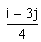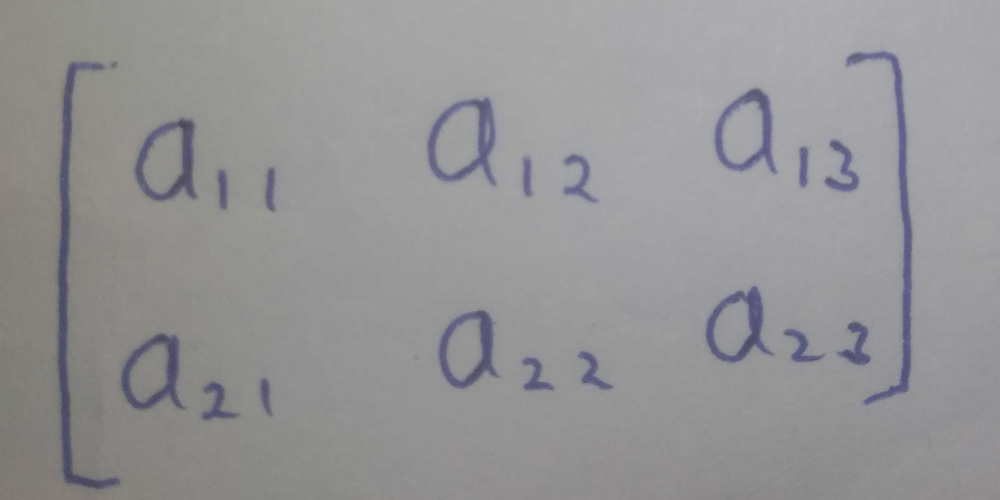Courses

# To construct a 2 x 3 matrix [ aij], such that aij = –The values that i and j can take are …….a)i = 1, 2, 3 ; j = 1, 2, 3b)i = 1, 2 ; j = 1, 2, 3c)i = 1, 2 ; j = 1, 2d)i = 1, 2, 3 ; j = 1, 2Correct answer is option 'B'. Can you explain this answer? Related Test: Test: Introduction To Matrices

## JEE Question

By Akriti Raj · 6 days ago ·JEE
Mohit Mittal answered May 08, 2020Kanan Aggarwal answered May 08, 2020
A